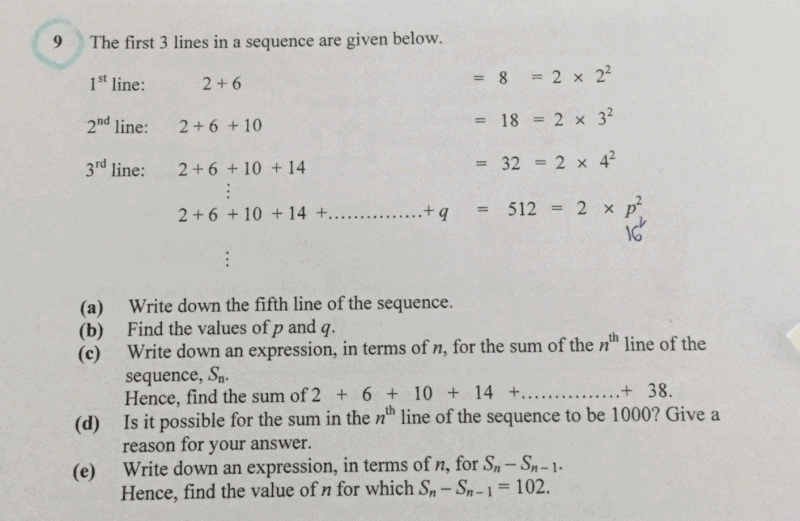# Questiona)2+6+10+14+18+22

b)q=16,p=2+15(4)=62

Note that the line is 16-1 while p=2+4(line number)

c)2+(n+1)^2

Last number of sequence is 38=2+9(4) so this is the 9th line. Sum will be 2+(10)^2=102

d) the sum will always be in the form 2+(n+1)^2. Since 1000-2=998 which is not a square, the sum cannot be 1000.

e) Sn-Sn-1 will cancel out all terms except the last term. Thus it will be equals to 2+4(n)

102-2=100=4(25) so n=25

0 Replies 0 Likes